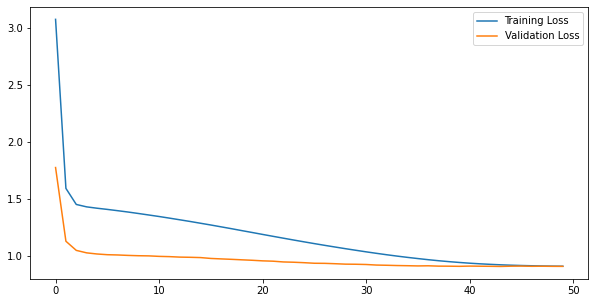# Matrix Factorization

Hello, thank you for the excellent explanation of the Matrix Factorization algorithm. Is there any specific reason that this has not been implemented in pytorch or tensorflow?

My result with pytorch and adding some regularization:

`Sampling = Random, test_size =0.1, Batch_size = 512, epoch_number = 50, L2 regularization = 0.0002, num_factors = 30, learning_rate=0.002, weight_decay=1e-5`

``````training loss is: 0.9014225415885448
val loss is: 0.9048249661922455
``````